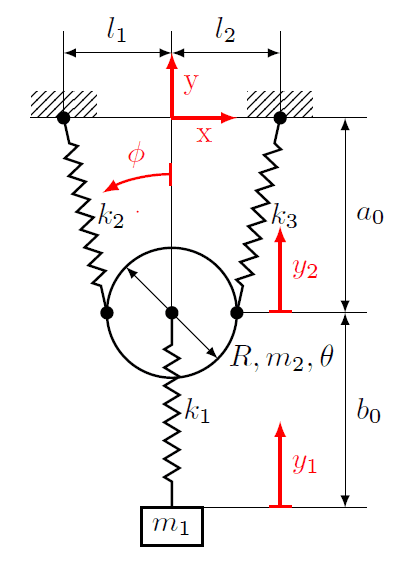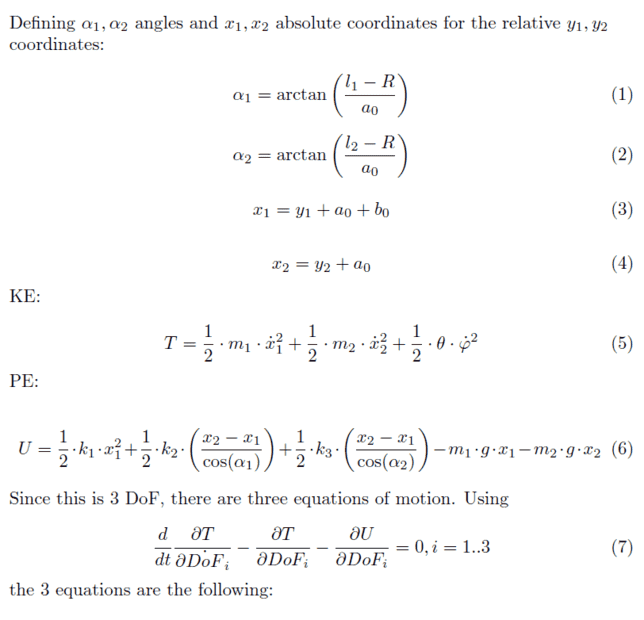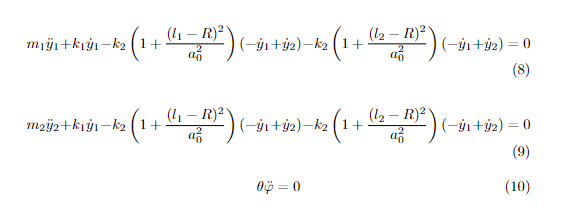# Vertical Spring System (Lagrangian)

andris0110
Homework Statement:
The problem states that for the vertical spring system below we give boundary conditions to the y positions of m1 and m2, so considering this I have to work out the equations of motion. The ring on the top is supposed to be moving only vertically.
Relevant Equations:
Lagrange equations of motion
I am trying to solve this and get the equations of motion using the Lagrangian method.
I could do all the steps but the equations (especially the third one) seems..weird.
What am I doing wrong? Sorry if the equations aren't in their simplest form, they are pulled straight from Wolfram Mathematica.
I tried to explain my work in a little more detail below:Thanks for the help in advance!

Homework Helper
Gold Member
2022 Award
Homework Statement:: The problem states that for the vertical spring system below we give boundary conditions to the y positions of m1 and m2, so considering this I have to work out the equations of motion. The ring on the top is supposed to be moving only vertically.
Relevant Equations:: Lagrange equations of motion

I am trying to solve this and get the equations of motion using the Lagrangian method.
I could do all the steps but the equations (especially the third one) seems..weird.
What am I doing wrong? Sorry if the equations aren't in their simplest form, they are pulled straight from Wolfram Mathematica.
I tried to explain my work in a little more detail below:
View attachment 316474

View attachment 316475
View attachment 316471

Thanks for the help in advance!
Hi @andris0110. Welcome to PF. Since no one has yet replied, here are a few comments.

If you haven’t yet read the guidelines for posting, it’s worth doing so: https://www.physicsforums.com/threads/homework-help-guidelines-for-students-and-helpers.686781/

There are some problems with what you have posted. Here are the first few I spotted before I gave up!

1. The original (complete/word-for-word-exact) question is missing. You have expressed the question in your own words. This may be OK but sometimes (often!) posters’ descriptions are incomplete and/or misinterpret the original question.

2. You say “the ring … is supposed to be moving only vertically”. But the ring appears to have the freedom to rotate about a horizontal axis through its centre while oscillating vertically. I’m guessing rotational motion is allowed and ##\theta## or ##\phi## is the angle of rotation.

3. The meanings of some parameters are unclear, e.g. ## \theta, \alpha_1 \alpha_2##. They should be shown on the diagram. (We can guess, but sometimes we'll guess wrongly.)

4. Are you using ‘x’ for both horizontal and vertical distances [Edit - and spring extension!]? That would be a dreadful source of confusion! Your potential energy equation (equation 6) seems to use ##x_1## and ##x_2## for the gravitational potential energy!

5. The kinetic energy term at the end of equation 5 appears to relate to rotational motion. But it makes no sense - it is dimensionally incorrect and doesn’t include the moment of inertia of the ring.

6. Rather than Wolfram Mathematica, you should formulate the various equations for yourself and include them in your posts. You should use LaTeX to format the equations here so we can read them easily. (You’ll get more replies/help if you create easy-to-read posts.) Here's the quick guide to LaTeX: https://www.physicsforums.com/help/latexhelp/. (This link is also below the bottom left corner of the edit window.)

I suggest taking a big step back. Your best bet might be to post a revised question/attempt with the above points addressed.

Edits: typo's corrected.

Last edited:
•PhDeezNutz, topsquark, TSny and 2 others